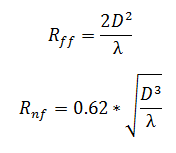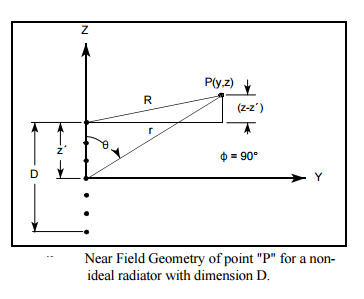# Antenna Near Field Distance Calculator

This CalcTown calculator calculates the near field ranges of an antenna. It determines the cross-over distance between the near and far field of an antenna and the limiting distances of the reactive near field, reactive radiating near field and radiating near field regions.

#### Result

m
mWhere,

D = Largest dimension of the antenna

f = Operating signal frequency

λ = Wavelength of the signal

Rff = Near field crossover distance (Fraunhoffer distance)

Rrn = Reactive near field limiting distance

Understanding the Antenna Near Field & Far Field Distances

The fields surrounding an antenna are divided into 3 main regions:

Reactive Near Field

The reactive near field ad the radiating near field. The reactive near field is the region where the fields are reactive i.e the E and H fields are out of phase by 90 degrees to each other. For propagating or radiating fields, the fields must be orthogonal to each other but in phase.